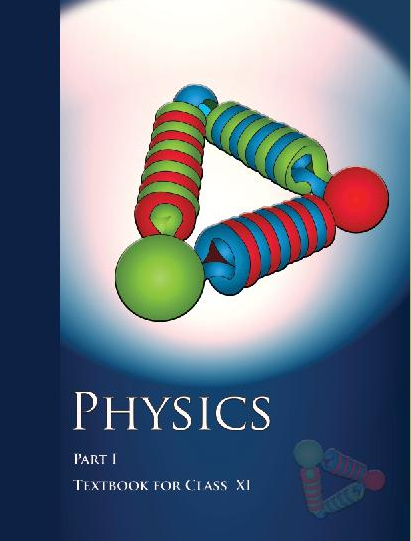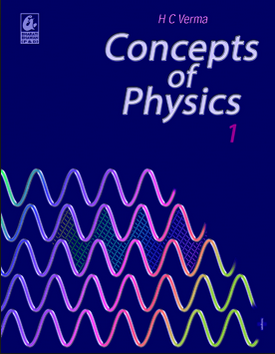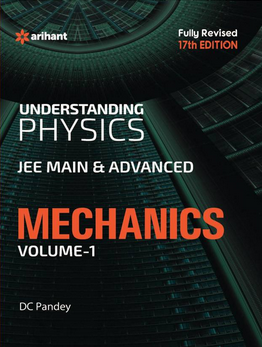# Experimental skills (Weightage 3%)   Share

### Topics from Experimental skills

• Identification of Diode, LED, Transistor, IC, Resistor, Capacitor from mixed collection of such items. (1 concepts)
• Using the multimeter (4 concepts)
• Characteristic curves (4 concepts)
• Potentiometer (3 concepts)
• Refractive index of a glass slab using a traveling microscope. (1 concepts)
• The plot of the angle of deviation vs angle of incidence for a triangular prism. (2 concepts)
• The focal length of Convex mirror, Concave mirror & Convex lens using parallax method. (2 concepts)
• Resistance and figure of merit of a galvanometer by half deflection method. (2 concepts)
• The resistivity of the material (1 concepts)
• Young's modulus of elasticity (1 concepts)
• Simple Pendulum (1 concepts)
• Metre Scale (1 concepts)
• The surface tension of water (1 concepts)
• The specific heat capacity (2 concepts)
• Plotting a cooling curve for the relationship between the temperature of a hot body and time. (1 concepts)
• The coefficient of Viscosity of a given viscous liquid (1 concepts)
• Young's modulus of elasticity (1 concepts)
• Screw gauge (1 concepts)
• Vernier calipers: (1 concepts)

### Important Books for Experimental skills

•••Exams
Articles
Questions